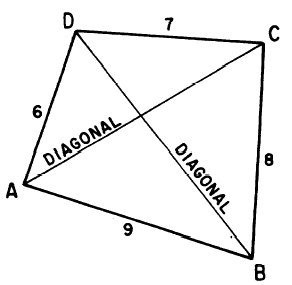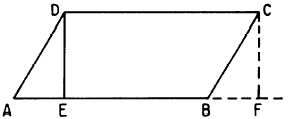Custom SearchQUADRILATERALS A QUADRILATERAL is a polygon with four sides. The parts of a quadrilateral are its sides, its four angles, and its two DIAGONALS. A diagonal is a straight line joining two alter AC and DB are the diagonals.Figure 17-13.-Parts of a quadrilateral. Perimeter and Area The perimeter of a quadrilateral is the sum of the lengths of its sides. For example, the perimeter of the quadrilateral in figure 17-13 is 30 units. The area of a quadrilateral can be found by dividing it into triangles and summing the areas of the triangles. However, the altitudes of the triangles are usually difficult to calculate un- less the quadrilateral has at least one pair of parallel sides. Parallelograms A PARALLELOGRAM is a quadrilateral in which the opposite sides are parallel. For example, in the parallelogram in figure 17-14, side AB is parallel to side CD. Furthermore, side BC is parallel to side AD.Figure 17-14.-A parallelogram. Since lines AB and CD are parallel, lines DE and CF (both perpendicular to line AF in figure 17-14) are equal. Angles DAE and CBF in figure 17-14 are equal, because a straight line cutting two parallel lines, such as AD and BC, forms equal angles with the parallel lines. Thus, triangles AED and BFC are equal, and line AD equals line BC. Therefore we have proved that the opposite sides of a parallelogram are equal. If all four of the sides are the same length, the parallelogram is a RHOMBUS. In addition to the equality of the opposite sides, the opposite angles of a parallelogram are also equal. For example, angle DAB equals angle BCD in figure 17-14, and angle ADC equals angle ABC. RECTANGLES AND SQUARES.-When all of the angles of a parallelogram are right angles, it is a RECTANGLE. A rectangle with all four of its sides the same length is a SQUARE. Thus a square is a rhombus having 90" angles. Every square is a rectangle, and every rectangle is a parallelogram. Notice that the reverse of this statement is not true. The area of a rectangle is found by multiplying its length times its width. Therefore, if each side of a square has length s, the area of the square is s2. Written as formulas, these areas are as follows:AREA.-The area of a parallelogram can be found by dividing it into rectangles and triangles. For example, in figure 17-14 the area of the parallelogram is the sum of the areas of triangle AED and figure EBCD. Since triangle AED is equal to triangle BFC, the sum of AED and EBCD is equal to the sum of BFC and EBCD. Thus the area of parallelogram ABCD is the same as the area of rectangle EFCD. Since the area of EFCD is DC multiplied by DE, and DC has the same length as AB, we conclude that the area of a parallelogram is the base as a formula, this is         A = ba or         A = bh, where h is height